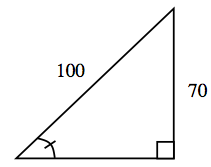### Home > INT2 > Chapter 7 > Lesson 7.1.3 > Problem7-41

7-41.

A $100$-foot long cable is attached $70$ feet up the side of a building. If it is pulled taut (i.e., there is no slack) and staked to the ground as far away from the building as possible, approximately what angle does the cable make with the ground?

1. $40.0^\circ$

1. $44.4^\circ$

1. $45.6^\circ$

1. $12.2^\circ$

$\sin(\theta)=\frac{\text{opposite}}{\text{hypotenuse}}$